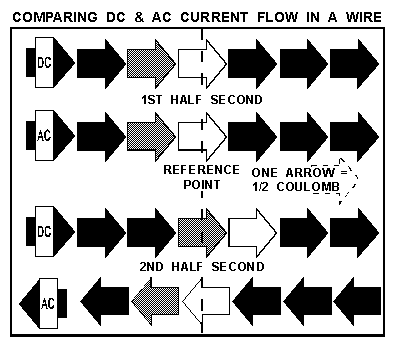Custom SearchAlternating CurrentVoltage WaveformCONCEPTS OF ALTERNATING CURRENT

LEARNING OBJECTIVES

Upon completion of this chapter you will be able to:

• State the differences between ac and dc voltage and current.
• State the advantages of ac power transmission over dc power transmission.
• State the "left-hand rule" for a conductor.
• State the relationship between current and magnetism.
• State the methods by which ac power can be generated.
• State the relationship between frequency, period, time, and wavelength.
• Compute peak-to-peak, instantaneous, effective, and average values of voltage and current.
• Compute the phase difference between sine waves.

CONCEPTS OF ALTERNATING CURRENT

All of your study thus far has been with direct current (dc), that is, current which does not change direction. However, as you saw in module 1 and will see later in this module, a coil rotating in a magnetic field actually generates a current which regularly changes direction. This current is called ALTERNATING CURRENT or ac.

AC AND DC

Alternating current is current which constantly changes in amplitude, and which reverses direction at regular intervals. You learned previously that direct current flows only in one direction, and that the amplitude of current is determined by the number of electrons flowing past a point in a circuit in one second. If, for example, a coulomb of electrons moves past a point in a wire in one second and all of the electrons are moving in the same direction, the amplitude of direct current in the wire is one ampere. Similarly, if half a coulomb of electrons moves in one direction past a point in the wire in half a second, then reverses direction and moves past the same point in the opposite direction during the next half-second, a total of one coulomb of electrons passes the point in one second. The amplitude of the alternating current is one ampere. The preceding comparison of dc and ac as illustrated. Notice that one white arrow plus one striped arrow comprise one coulomb.Q.1 Define direct current.
Q.2 Define alternating current.

DISADVANTAGES OF DC COMPARED TO AC

When commercial use of electricity became wide-spread in the United States, certain disadvantages in using direct current in the home became apparent. If a commercial direct-current system is used, the voltage must be generated at the level (amplitude or value) required by the load. To properly light a 240-volt lamp, for example, the dc generator must deliver 240 volts. If a 120-volt lamp is to be supplied power from the 240-volt generator, a resistor or another 120-volt lamp must be placed in series with the 120-volt lamp to drop the extra 120 volts. When the resistor is used to reduce the voltage, an amount of power equal to that consumed by the lamp is wasted.

Another disadvantage of the direct-current system becomes evident when the direct current (I) from the generating station must be transmitted a long distance over wires to the consumer. When this happens, a large amount of power is lost due to the resistance (R) of the wire. The power loss is equal to I2R. However, this loss can be greatly reduced if the power is transmitted over the lines at a very high voltage level and a low current level. This is not a practical solution to the power loss in the dc system since the load would then have to be operated at a dangerously high voltage. Because of the disadvantages related to transmitting and using direct current, practically all modern commercial electric power companies generate and distribute alternating current (ac).

Unlike direct voltages, alternating voltages can be stepped up or down in amplitude by a device called a TRANSFORMER. (The transformer will be explained later in this module.) Use of the transformer permits efficient transmission of electrical power over long-distance lines. At the electrical power station, the transformer output power is at high voltage and low current levels. At the consumer end of the transmission lines, the voltage is stepped down by a transformer to the value required by the load. Due to its inherent advantages and versatility, alternating current has replaced direct current in all but a few commercial power distribution systems.

Q.3 What is a disadvantage of a direct-current system with respect to supply voltage?
Q.4 What disadvantage of a direct current is due to the resistance of the transmission wires?
Q.5 What kind of electrical current is used in most modern power distribution systems?Integrated Publishing, Inc. - A (SDVOSB) Service Disabled Veteran Owned Small Business